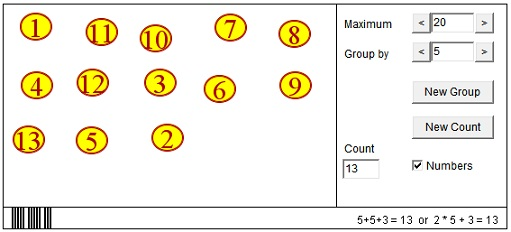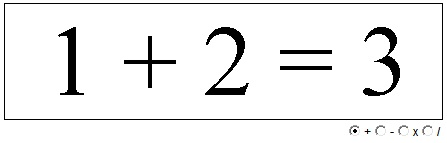# Counting: The Beginning of Mathematics

Counting various quantities is the foremost human activity in which children engage beginning at a very tender age. The main property of counting is so fundamental to our perception of quantity that it is seldom enunciated explicitly. The purpose of counting is to assign a numeric value to a group of objects. What makes counting possible? A simple fact that such a value exists. However we go about counting the number of eggs in a basket the result is always the same. We often count them a couple of times to see that we got the right number.

This fact can't be overemphasized. By merely verbalizing it we make a first step to understanding what mathematics is all about. This is because from this fact alone one may deduce a variety of conclusions. Here then the First Principle of Counting

The result of counting objects in a group does not depend on the manner in which the process of counting is conducted.

What can be deduced from this statement? Assume we are given two groups of objects. Remove an object from one group and place it into the other one. From the First Principle of Counting (sic!), this operation does not change the totality of objects in the two groups. Is that that obvious? Of course. But who said that mathematics is difficult?

To obtain the total quantity in the two groups, we agree to first count one group and then continue counting the remaining one. From the First Principle of Counting it follows that if we start counting with the second group and then move on to the first one, the result will be exactly the same. In mathematical terms, a + b = b + a - commutativity of addition. Why do we need special terms like addition and commutativity and symbols like "+" and "="? Because of the convenience of having short descriptives for very common situations and to underscore the generality of our understanding: the nature of objects in the groups is absolutely inconsequential. The only thing that matters is the quantities involved. Quantities are described either verbally or by number symbols (known as numerals), while variables (or generic names) substitute for unspecified quantities.

It appears that addition is intrinsically related to counting. Lewis Carroll's Red Queen would agree:

"What's one and one and one and one and one and one and one and one and one and one?"
"I don't know, " said Alice, "I lost count."
"She can't do addition," said the Red Queen.

But repeatedly adding 1 is a very primitive form of addition which is also not very useful. While typing the quote I also lost the count several times. It is much easier to count objects by grouping them into small groups and later adding up the quantities associated with every group. If all the groups have the same amount of objects, we will discern the relevance of another arithmetic operation - multiplication - to counting. For example, the mathematical notation for "2 and 2 and 2" is "3 times 2" or, symbolically, 3·2. 3·2 is thus the same as "1 and 1 and 1 and 1 and 1 and 1" which is denoted as 6: 3·2 = 6. Sometimes, we can't split objects evenly into smaller groups. For example, splitting 7 into groups of 3 objects, we get 2 groups and 1 leftover object. In this case, addition and multiplication combine in the mathematical notation: 2·3 + 1 = 7. This is how counting leads to the idea of division and even of division with remainder. 3·2 = 6 means not only that three groups of 2 elements each total 6 elements together, but also that a group of 6 elements can be divided into 3 groups of 2 elements each. The second part being a mere paraphrase of the first part of the previous statement. When we attempt to divide 7 into two groups we get two groups of three objects and 1 additional element. 7 is not divided evenly by 2.

Following is an applet that helps explore these concepts. See that this is indeed possible to count and group objects in many different ways that always lead to the same result. Observe the mathematical notations used to describe various groupings of objects. Count objects by clicking on them in turn.

### If you are reading this, your browser is not set to run Java applets. Try IE11 or Safari and declare the site https://www.cut-the-knot.org as trusted in the Java setup.What if applet does not run?

The next applet is a little more abstract. Counting is a useful craft but we already know that addition and multiplication may speed it up, often considerably. In this applet, you may click on each of the three numbers. Clicking a little to the right of its central line will increase the number by one. Clicking a little to the left of the number decreases the number by 1. The mathematical symbol ">" stands for more than, "<" stands for less than. You start with the common fact 1 + 1 = 2 which simply says that 2 denotes the number of elements in a group that is counted as "one and one", or which consists of two smaller groups each having 1 element. If you add an element to either of the smaller groups (or numbers on the left), to preserve the equality, you must also add a 1 on the right. (To change numbers faster, you can drag the mouse on the left or right of their central line.)

### If you are reading this, your browser is not set to run Java applets. Try IE11 or Safari and declare the site https://www.cut-the-knot.org as trusted in the Java setup.What if applet does not run?

Finally, here is an applet that is a variation on a problem with two groups. Say, you have apples and peaches. All together there are 12 fruits. There are two more apples than peaches. How many of each fruit are there? Note that the problem does not always have a solution. Try to think of when it does.

### If you are reading this, your browser is not set to run Java applets. Try IE11 or Safari and declare the site https://www.cut-the-knot.org as trusted in the Java setup.What if applet does not run?Prof. W. McWorter was kind enough to share his experiences teaching counting to young students.

In his book Tracking The Automatic Ant (Springer, 1998), David Gale tells the following story:

Once upon a time there was a little girl named Clara who was barely three years old and had just learned how to count. She could tell how many chairs were in the living room and the number of steps from the front porch. One day her father decided to test her. "Look," he said, "I've brought you these four lollipops," but he handed her only three. Clara took the lollipops and dutifully counted, "One, two, four." Then she looked up a bit puzzled and asked, "Where's the third one?"•